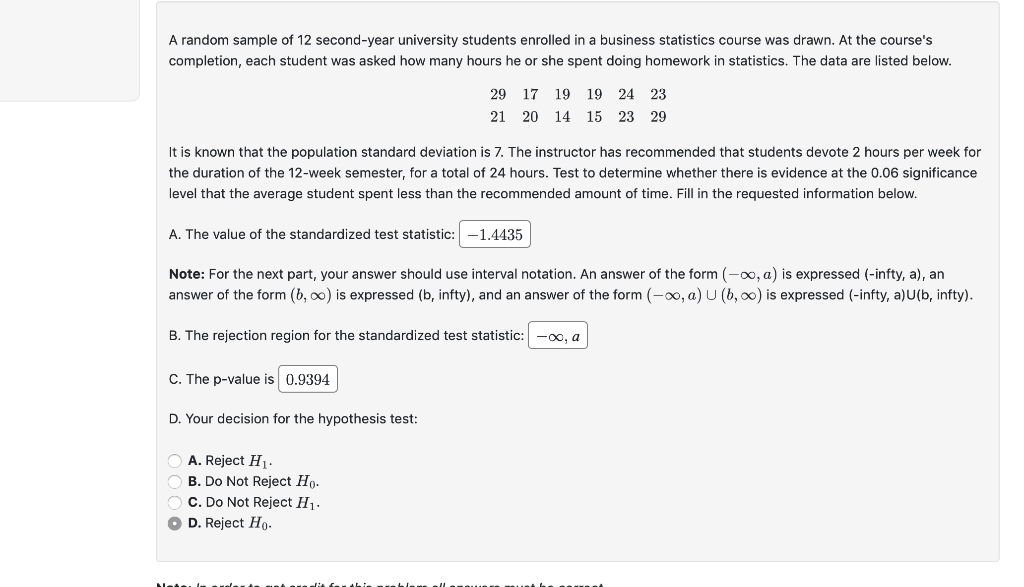# (Solved): A random sample of 12 second-year university students enrolled in a business ...​​​​​​​

A random sample of 12 second-year university students enrolled in a business statistics course was drawn. At the course's completion, each student was asked how many hours he or she spent doing homework in statistics. The data are listed below. It is known that the population standard deviation is 7 . The instructor has recommended that students devote 2 hours per week for the duration of the 12-week semester, for a total of 24 hours. Test to determine whether there is evidence at the 0.06 significance level that the average student spent less than the recommended amount of time. Fill in the requested information below. A. The value of the standardized test statistic: Note: For the next part, your answer should use interval notation. An answer of the form is expressed (-infty, a), an answer of the form is expressed ( , infty), and an answer of the form is expressed (-infty, a) , infty). B. The rejection region for the standardized test statistic: C. The p-value is D. Your decision for the hypothesis test: A. Reject . B. Do Not Reject . C. Do Not Reject . D. Reject .

We have an Answer from Expert